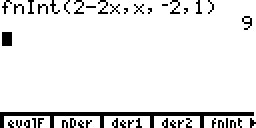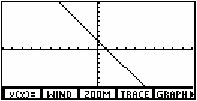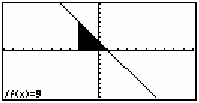# Knowledge Base

## Solution 10580: Finding the Area Below a Function Using a TI-86.

### How do I find the area below a function using a TI-86?

To find the area below a function using a TI-86, the command FnInt or the graphing integral function can be used. The FnInt function, which finds a numerical approximation for a definite integral, uses the following syntax:

FnInt(expression, variable name, lower limit, upper limit)

NOTE: To access this function, press [2nd] [CALC] [F5].

Example: What is the area under the function y = 2 - 2x between -2 and 1?

• Press [2nd] [CALC] [F5].

• Input 2-2x,x,-2,1

• Press [)] [ENTER]. The calculator should display 9 as the solution.The area below the function can also be found by using the Graph screen. Please follow the steps below:

• Press [GRAPH] [F1] to access the y= editor.

• Enter the function 2-2x.

• Press [ENTER].

• Press [2nd] [F5] to display the graph.• Press [MORE].

• Press [F1] for MATH.

• Press [F3] to select the integral function.

• For "Lower Limit", input -2 and press [ENTER].

• For "Upper Limit", input 1 and press [ENTER].

The graph is displayed with the region shaded between -2 and 1.